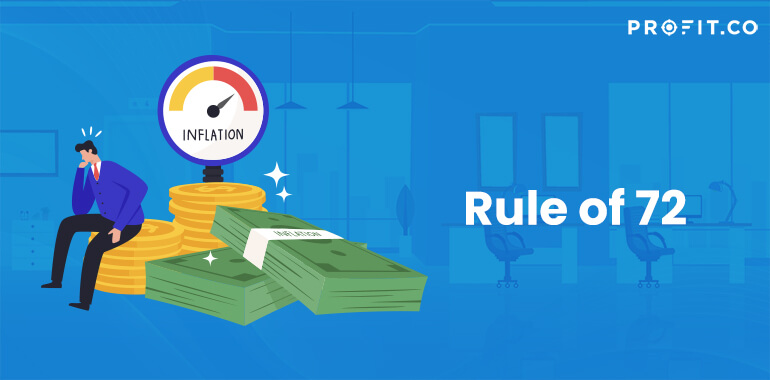Category: Finance KPIs.

If you want to see for how long you have to invest your money in order to double it, with a given interest rate, then you will most certainly use the rule of 72. Basically, this is used to determine the number of years in which your money will double in value with compounding interest.

Before making a certain investment, investors will use the rule of 72 in order to see the differences between two investments and, therefore, choose the best one to invest in – namely, the one that has higher chances of doubling his or her money, preferably in a shorter time period.

Naturally, an investor will probably go for the investment that will return a profit in a short period of time, so that he or she can then use the profit in order to make another investment and so on.

Understanding the Rule of 72

However, the rule of 72 isn’t of use only to investors – everyone can use it in order to determine various things. For example, one could determine the amount of money they are going to have in a retirement account, or how much their share will be worth, in a mutual fund, after a five-year period.

In short, the rule of 72 can be seen as a value calculator that will calculate, of course, the value your investment will have in the future.

This formula is preferred over the investment equation for compounding interest because the latter is quite long and complicated. It works as a great shortcut and can be used as a base for any of your investment.

The Formula of Rule of 72

The rule of 72 has three formulas that can be applied, depending on the information that you want to find out. The first formula works a bit different from the other two, as the result will always be equal to 72. So, in the first case, we will multiply the investment rate by the number of years that will be invested – the end product will always be equal to 72.

Working a bit around the aforementioned formula we find out the number of years we have to invest in a certain investment or the investment rate desired in order to eventually double the initial amount of money invested.

If we want to know the number of years we’ll have to invest, we have to divide 72 by the annual investment rate. If we want to find out the investment rate that’s required in order to double our money, we’ll have to divide 72 by the number of years invested.

Final Thoughts

Important for you to remember is that the interest used in the formula must be compounded annually. Therefore, investments that have quarterly compound or semi-annual interest can’t be calculated using this formula.

Moreover, you must keep in mind that the formulas above do not take into account anything that might change the values of one of the equation’s terms – for example, future inflation rates might change, and therefore change the outcome of your investment as well.

However, the rule of 72 can also be used in order to determine the effects the inflation will have on your money.

## Related Articles

• ### Sharpe Ratio

In order to be sound in your financial goals, you need to have a great source of knowledge on every... Read more